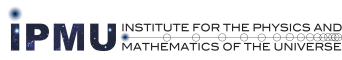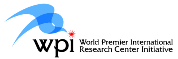### IPMU Komaba Seminar

 Lecturer: Jean-Michel Bismut (Univ. Paris-Sud, Orsay) Date: Lecture 1: May 12, from 17:00 - 18:30 "The hypoelliptic Laplacian" Lecture 2: May 19, from 17:00 - 18:30 "A survey of Quillen metrics" Place: Room 002, Mathematical Sciences Building, Komaba Campus, The University of Tokyo This Lecture can be attended in room 623, ICRR (May 12) and room 031, General Research Building (May 19), Kashiwa Campus using TV conference system. Abstract: "The hypoelliptic LaplacianA survey of Quillen metrics" Let X be a compact Riemannian manifold. The Laplace Beltrami operator -ΔX, or more generally the Hodge Laplacian square(X), is an elliptic second order self adjoint operator on X. We will explain the construction of a deformation of the elliptic Laplacian to a family of hypoelliptic operators acting on the total space of the cotangent bundle X. These operators depend on a parameter b > 0, and interpolate between the Hodge Laplacian (the limit as b → 0) and the geodesic flow (the limit as b → + ∞). Actually, the deformed Laplacian is associated with an exotic Hodge theory on the total space of the cotangent bundle, in which the standard L2 scalar product on forms is replaced by a symmetric bilinear form of signature ( ∞, ∞). This deformation can be understood as a version of the Witten deformation on the loop space associated with the energy functional. From a probabilistic point of view, the deformed Laplacian corresponds to a Langevin process. The above considerations can also be used in complex geometry, in which the Dolbeault cohomology is considered instead of the Rham cohomology. Results obtained with Gilles Lebeau on the analysis of the hypoelliptic Laplacian will also be presented, as well as applications to analytic torsion. "A survey of Quillen metrics" In this lecture, I will survey basic results on Quillen metrics. Indeed let X be a complex K″ahler manifold, and let E be a holomorphic Hermitian vector bundle on X. Let λ be the complex line which is the determinant of the cohomology of E. The Quillen metric is a metric on the line λ, which one obtains using a spectral invariant of the Hodge Laplacian, the Ray-Singer analytic torsion. The Quillen metrics have a number of remarkable properties. Among them the curvature theorem says that when one considers a family of such X, the curvature of the holomorphic Hermitian connection on λ is given by a formula of Riemann-Roch-Grothendieck type. I will explain some of the ideas which go into the proof of these properties, which includes Quillen's superconnections. Note: This seminar is organized by Institute for the Physics and Mathematics of the Universe (IPMU) and Graduate School of Mathematical Sciences, The University of Tokyo. IPMU Komaba Seminar Web Page http://faculty.ms.u-tokyo.ac.jp/~topology/IPMU/index.html